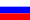# Vestnik КRAUNC. Fiz.-Mat. nauki. 2022. vol. 39. no. 2. P. 20-31. ISSN 2079-6641

Contents of this issue

Read Russian VersionMSC 35K05, 35K15

Research Article

On the control problem associated with the heating process in the bounded domain

F. N. Dekhkonov¹²

¹National University of Uzbekistan named after Mirzo Ulugbek, 100174, University str., 4b, Tashkent, Uzbekistan
²Institute of Mathematics named after V. I. Romanovskiy, Academy of Sciences of the Republic of Uzbekistan, 100174, University str., 4b, Tashkent, Uzbekistan

E-mail: f.n.dehqonov@mail.ru

The initial-boundary problem for the heat conduction equation inside a bounded domain is considered. It is supposed that on the boundary of this domain the heat exchange takes place according to Newton’s law. The control parameter is equal to the magnitude of output of hot air and is defined on a givenmpart of the boundary. Then we determined the dependence T(θ) on the parameters of the temperature process when θ is close to critical value.

Key words: heat conduction equation, admissible control, initial-boundary value problem, integral equation.

DOI: 10.26117/2079-6641-2022-39-2-20-31

Original article submitted: 01.07.2022

Revision submitted: 10.08.2022

For citation. Dekhkonov F. N. On the control problem associated with the heating process in the bounded domain. Vestnik KRAUNC. Fiz.-mat. nauki. 2022, 39: 2, 20-31. DOI: 10.26117/2079-6641-2022-39-2-20-31

Competing interests. The author declares no conflicts of interest with respect to authorship and publication.

Contribution and responsibility. The author has contributed to this article. The author is solely responsible for providing the final version of the article for publication.

Acknowledgements. The author is grateful to Academician Sh. A. Alimov for his valuable comments.

References

1. Albeverio S., Alimov Sh. A. On a time-optimal control problem associated with the heat exchange process, Applied Mathematics and Optimization, 2008. vol. 57, no. 1, pp. 58–68.
2. Alimov Sh. A., Dekhkonov F. N. On a control problem associated with fast heating of a thin rod, Bulletin of National University of Uzbekistan: Mathematics and Natural Sciences, 2019. vol. 2, no. 1, pp. 1–14.
3. Alimov Sh. A., Dekhkonov F. N. On the time-optimal control of the heat exchange process, Uzbek
Mathematical Journal, 2019. no. 2, pp. 4–17.
4. Altmüller A., Grüne L. Distributed and boundary model predictive control for the heat equa- tion, Technical report, University of Bayreuth, Department of Mathematics, 2012.
5. Chen N., Wang Y., Yang D. Time–varying bang–bang property of time optimal controls for heat equation and its applications, Syst. Control Lett, 2018. vol. 112, pp. 18–23.
6. Egorov Yu. V. Optimal control in Banach spaces, Dokl. Akad. Nauk SSSR, 1963. vol. 150, pp. 241– 244 (In Russian).
7. Fattorini H. O. Time-optimal control of solutions of operational differential equations, SIAM J. Con- trol, 1964. vol. 2, pp. 49–65.
8. Fattorini H. O. Time and norm optimal controls: a survey of recent results and open problems, Acta Math. Sci. Ser. B Engl. Ed., 2011. vol. 31, pp. 2203–2218.
9. Friedman A. Optimal control for parabolic equations, J. Math. Anal. Appl., 1967. vol. 18, pp. 479– 491.
10. Dekhkonov F. N. On time-optimal control problem associated with parabolic equation, Bulletin of National University of Uzbekistan, 2021. vol. 4, no. 1, pp. 54–63.
11. Dekhkonov F. N. On a time-optimal control of thermal processes in a boundary value problem, Lobachevskii journal of mathematics, 2022. vol. 43, no. 1, pp. 192–198 DOI:10.1134/S1995080222040096.
12. Fursikov A. V. Optimal Control of Distributed Systems: Math. Soc., Providence, Rhode Island, 2000.
13. Ladyzhenskaya O. A., Solonnikov V. A., Uraltseva N. N. Linear and Quasi-Linear Equations of Parabolic Type. Moscow: Nauka, 1967 (In Russian).
14. Lions J. L. Contróle optimal de systèmes gouvernés par deséquations aux dérivées partielles. Dunod Gauthier-Villars: Paris, 1968.
15. Miranda C. Equazioni alle derivate parziali di tipo ellittico. Springer Verlag: Berlin-Göttingen- Heidelberg, 1955.
16. Tikhonov A. N., Samarsky A. A. Equations of Mathematical Physics. N. Chelmsford: Courier Corporation, 2013. 800 pp.
17. Vladimirov V. S. Equations of Mathematical Physics. Marcel Dekker: New York, 1971.

Dekhkonov Farrukh Nuriddin ugli – Ph.D. (Phys. & Math.), National University of Uzbekistan named after M.Ulugbek, Tashkent, Uzbekistan, ORCID 0000-0003-4747-8557.